# Exercise PK15 - Toxicokinetics

### Background

• Structure - Non Compartmental Analysis

• Route of administration - Oral

• Dosage Regimen - 10, 56 and 320 μmol/kg/day

• Number of Subjects - 30

• Study Period - 12 month safety study in male and female dogs

### Learning Outcome

Application of Non Compartmental data analysis

### Objectives

• To analyze the kinetics by means of means of Non-compartmental Analysis (NCA)

• To analyze the exposure to test comounds by means of Cmax and AUC.

### Libraries

call the "necessary" libraries to get start

using Pumas
using PumasTutorials
using Plots
using CSV
using StatsPlots


### Import the Dataset

Import the dataset and perform data-wrangling to make the datset ready for read_nca

• Time = hrs

• Conc = μmol/kg

• Dose_grp = μmol/kg

• Period = weeks

data = DataFrame(CSV.File(joinpath(dirname(pathof(PumasTutorials)), "..", "data", "PKPDDataAnalysisBook", "pk15data.csv")))

##delete the first row which contains untits
delete!(data, )

##sort by Subject ID, Period and Time
sort!(data, [:Subject_ID,:Period,:Time])

##remove rows containing other Periods and Dose_grp
filter!(x -> x.Period in ["11","26", "52"], data)
filter!(x -> x.Dose_grp in ["10","56","320"], data)

## Convert the colums to necesaary types for input
data[:Conc] = parse.(Float64,data[:Conc])
data[:Time] = parse.(Float64,data[:Time])
data[:Period] = parse.(Int64,data[:Period])
data[:Dose_grp] = parse.(Int64,data[:Dose_grp])

##sort by Subject ID, Period and Time
sort!(data, [:Subject_ID,:Period,:Time])

## Add new column for Route of administration - extravascular
data[!,:route] .= "ev"


### Plots

##### Period - Week 11, Males
male_11 = filter(x -> x.Period == 11, data)
filter!(x -> x.Gender == "male", male_11)
male_11_gr1 = filter(x -> x.Dose_grp == 10, male_11)
male_11_gr2 = filter(x -> x.Dose_grp == 56, male_11)
male_11_gr3 = filter(x -> x.Dose_grp == 320, male_11)

@df male_11_gr1 plot(:Time, :Conc, yaxis=:log,
title = "Period (Week) = 11, male", xlabel = "Time (hr)", ylabel = "Concentration (uM)",
xticks = [0,5,10,15,20,25], xlims = (0,25), yticks = [0.01,0.1,1,10,100], ylims = (0.01,100),
group=:Subject_ID, colour=[:red], label=false)
@df male_11_gr2 plot!(:Time, :Conc, yaxis=:log, group=:Subject_ID, colour=[:blue], label=false)
@df male_11_gr3 plot!(:Time, :Conc, yaxis=:log, group=:Subject_ID, colour=[:grey], label=false)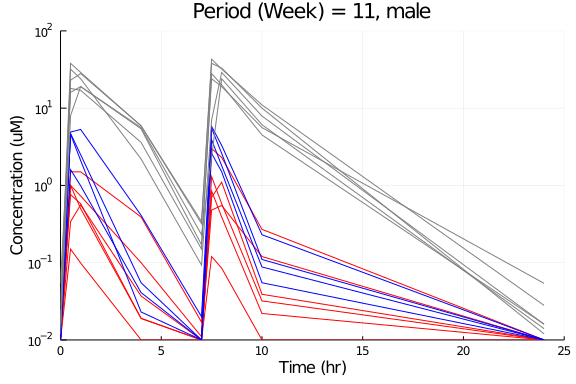##### Period - Week 26, Males
male_26 = filter(x -> x.Period == 26, data)
filter!(x -> x.Gender == "male", male_26)
male_26_gr1 = filter(x -> x.Dose_grp == 10, male_26)
male_26_gr2 = filter(x -> x.Dose_grp == 56, male_26)
male_26_gr3 = filter(x -> x.Dose_grp == 320, male_26)

@df male_26_gr1 plot(:Time, :Conc, yaxis=:log,
title = "Period (Week) = 26, male", xlabel = "Time (hr)", ylabel = "Concentration (uM)",
xticks = [0,5,10,15,20,25], xlims = (0,25), yticks = [0.01,0.1,1,10,100], ylims = (0.01,100),
group=:Subject_ID, colour=[:red], label=false)
@df male_26_gr2 plot!(:Time, :Conc, yaxis=:log, group=:Subject_ID, colour=[:blue], label=false)
@df male_26_gr3 plot!(:Time, :Conc, yaxis=:log, group=:Subject_ID, colour=[:grey], label=false)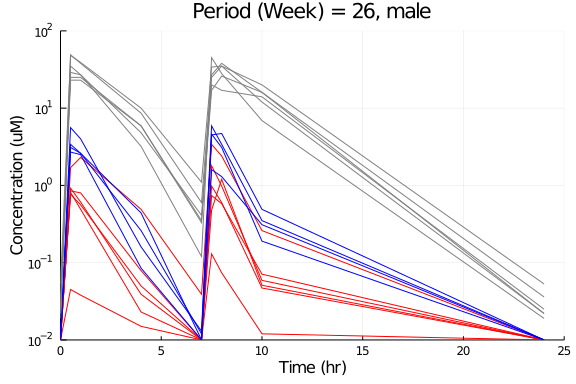##### Period - Week 52, Males
male_52 = filter(x -> x.Period == 52, data)
filter!(x -> x.Gender == "male", male_52)
male_52_gr1 = filter(x -> x.Dose_grp == 10, male_52)
male_52_gr2 = filter(x -> x.Dose_grp == 56, male_52)
male_52_gr3 = filter(x -> x.Dose_grp == 320, male_52)

@df male_52_gr1 plot(:Time, :Conc, yaxis=:log,
title = "Period (Week) = 52, male", xlabel = "Time (hr)", ylabel = "Concentration (uM)",
xticks = [0,5,10,15,20,25], xlims = (0,25), yticks = [0.01,0.1,1,10,100], ylims = (0.01,100),
group=:Subject_ID, colour=[:red], label=false)
@df male_52_gr2 plot!(:Time, :Conc, yaxis=:log, group=:Subject_ID, colour=[:blue], label=false)
@df male_52_gr3 plot!(:Time, :Conc, yaxis=:log, group=:Subject_ID, colour=[:grey], label=false)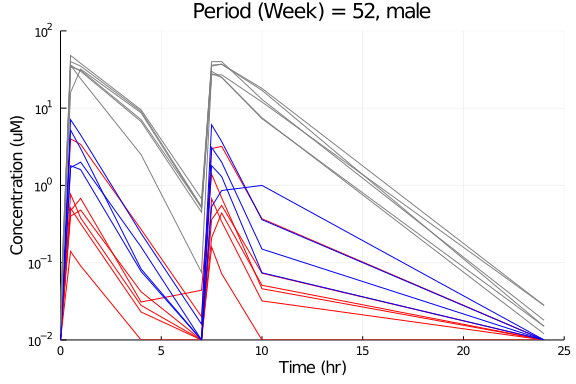##### Period - Week 11, Females
female_11 = filter(x -> x.Period == 11, data)
filter!(x -> x.Gender == "female", female_11)
female_11_gr1 = filter(x -> x.Dose_grp == 10, female_11)
female_11_gr2 = filter(x -> x.Dose_grp == 56, female_11)
female_11_gr3 = filter(x -> x.Dose_grp == 320, female_11)

@df female_11_gr1 plot(:Time, :Conc, yaxis=:log,
title = "Period (Week) = 11, female", xlabel = "Time (hr)", ylabel = "Concentration (uM)",
xticks = [0,5,10,15,20,25], xlims = (0,25), yticks = [0.01,0.1,1,10,100], ylims = (0.01,100),
group=:Subject_ID, colour=[:red], label=false)
@df female_11_gr2 plot!(:Time, :Conc, yaxis=:log, group=:Subject_ID, colour=[:blue], label=false)
@df female_11_gr3 plot!(:Time, :Conc, yaxis=:log, group=:Subject_ID, colour=[:grey], label=false)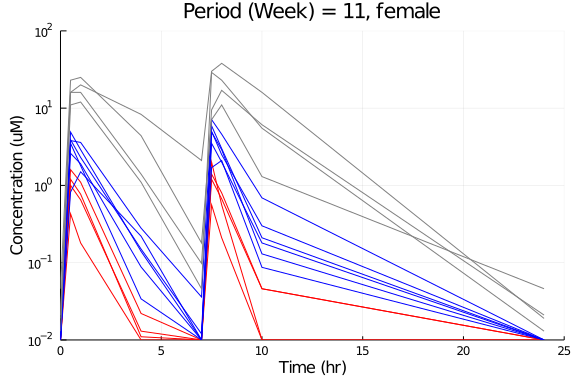##### Period - Week 26, Females
female_26 = filter(x -> x.Period == 26, data)
filter!(x -> x.Gender == "female", female_26)
female_26_gr1 = filter(x -> x.Dose_grp == 10, female_26)
female_26_gr2 = filter(x -> x.Dose_grp == 56, female_26)
female_26_gr3 = filter(x -> x.Dose_grp == 320, female_26)

@df female_26_gr1 plot(:Time, :Conc, yaxis=:log,
title = "Period (Week) = 26, female", xlabel = "Time (hr)", ylabel = "Concentration (uM)",
xticks = [0,5,10,15,20,25], xlims = (0,25), yticks = [0.01,0.1,1,10,100], ylims = (0.01,100),
group=:Subject_ID, colour=[:red], label=false)
@df female_26_gr2 plot!(:Time, :Conc, yaxis=:log, group=:Subject_ID, colour=[:blue], label=false)
@df female_26_gr3 plot!(:Time, :Conc, yaxis=:log, group=:Subject_ID, colour=[:grey], label=false)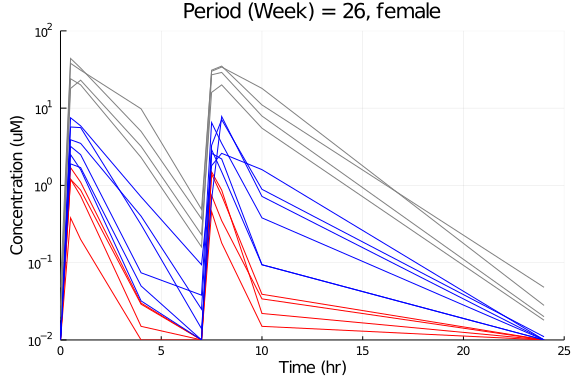##### Period - Week 52, Females
female_52 = filter(x -> x.Period == 52, data)
filter!(x -> x.Gender == "female", female_52)
female_52_gr1 = filter(x -> x.Dose_grp == 10, female_52)
female_52_gr2 = filter(x -> x.Dose_grp == 56, female_52)
female_52_gr3 = filter(x -> x.Dose_grp == 320, female_52)

@df female_52_gr1 plot(:Time, :Conc, yaxis=:log,
title = "Period (Week) = 52, female", xlabel = "Time (hr)", ylabel = "Concentration (uM)",
xticks = [0,5,10,15,20,25], xlims = (0,25), yticks = [0.01,0.1,1,10,100], ylims = (0.01,100),
group=:Subject_ID, colour=[:red], label=false)
@df female_52_gr2 plot!(:Time, :Conc, yaxis=:log, group=:Subject_ID, colour=[:blue], label=false)
@df female_52_gr3 plot!(:Time, :Conc, yaxis=:log, group=:Subject_ID, colour=[:grey], label=false)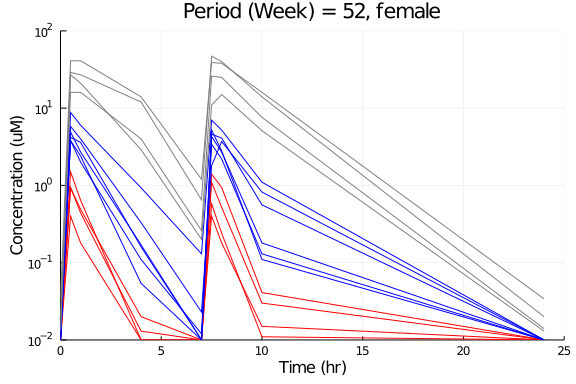### NCA Analysis

Perform the NCA Analysis

timeu = u"hr"
concu = u"μmol/kg"
amtu  = u"μmol/kg"

nca_analysis = read_nca(data,
id    = :Subject_ID,
time  = :Time,
conc  = :Conc,
route = :route,
amt   = :Dose,
group = [:Period,:Gender],
timeu = timeu,
concu = concu,
amtu  = amtu)

report_nca  = NCAReport(nca_analysis, sigdig=3)


90 rows × 41 columns (omitted printing of 33 columns)

idPeriodGenderdoseamttlagtmaxcmaxtlast
Int64StringStringQuantit…Quantit…Quantit…Quantit…Quantit…
1711female10 μmol kg⁻¹0.0 hr7.5 hr2.0 μmol kg⁻¹24.0 hr
2911female10 μmol kg⁻¹0.0 hr7.5 hr1.4 μmol kg⁻¹24.0 hr
31111female56 μmol kg⁻¹0.0 hr7.5 hr5.0 μmol kg⁻¹24.0 hr
41311female56 μmol kg⁻¹0.0 hr0.5 hr4.9 μmol kg⁻¹24.0 hr
51511female56 μmol kg⁻¹0.0 hr7.5 hr7.1 μmol kg⁻¹24.0 hr
61711female320 μmol kg⁻¹0.0 hr1.0 hr25.0 μmol kg⁻¹24.0 hr
71911female320 μmol kg⁻¹0.0 hr8.0 hr38.0 μmol kg⁻¹24.0 hr
82711female10 μmol kg⁻¹0.0 hr0.5 hr1.2 μmol kg⁻¹24.0 hr
92911female10 μmol kg⁻¹0.0 hr7.5 hr0.56 μmol kg⁻¹24.0 hr
103111female56 μmol kg⁻¹0.0 hr7.5 hr5.9 μmol kg⁻¹24.0 hr
113311female56 μmol kg⁻¹0.0 hr7.5 hr3.5 μmol kg⁻¹24.0 hr
123511female56 μmol kg⁻¹0.0 hr0.5 hr3.5 μmol kg⁻¹24.0 hr
133711female320 μmol kg⁻¹0.0 hr7.5 hr29.0 μmol kg⁻¹24.0 hr
143911female320 μmol kg⁻¹0.0 hr8.0 hr17.0 μmol kg⁻¹24.0 hr
15611male10 μmol kg⁻¹0.0 hr7.5 hr1.3 μmol kg⁻¹24.0 hr
16811male10 μmol kg⁻¹0.0 hr0.5 hr1.0 μmol kg⁻¹24.0 hr
171011male10 μmol kg⁻¹0.0 hr8.0 hr1.1 μmol kg⁻¹24.0 hr
181211male56 μmol kg⁻¹0.0 hr7.5 hr5.5 μmol kg⁻¹24.0 hr
191411male56 μmol kg⁻¹0.0 hr7.5 hr2.6 μmol kg⁻¹24.0 hr
201611male320 μmol kg⁻¹0.0 hr7.5 hr38.0 μmol kg⁻¹24.0 hr
211811male320 μmol kg⁻¹0.0 hr7.5 hr28.0 μmol kg⁻¹24.0 hr
222011male320 μmol kg⁻¹0.0 hr8.0 hr24.0 μmol kg⁻¹24.0 hr
232611male10 μmol kg⁻¹0.0 hr7.5 hr3.0 μmol kg⁻¹24.0 hr
242811male10 μmol kg⁻¹0.0 hr0.5 hr0.15 μmol kg⁻¹24.0 hr